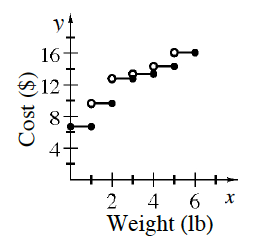### Home > INT2 > Chapter 10 > Lesson 10.1.1 > Problem10-9

10-9.

Andres is mailing some presents to his cousins.The rates for sending packages are shown in the table.

 Weight not above (lb) Cost $(\)$ $1$ $6.73$ $2$ $9.64$ $3$ $12.78$ $4$ $13.38$ $5$ $14.33$ $6$ $16.08$
1. What type of function would best represent this situation?

It is a step function.

2. Sketch a graph of the cost of a package by weight.

It is a step function.3. Andres wants to mail one present that weighs $2.2$ pounds and another present that weighs $1.8$ pounds. Should he send them in one package or two separate packages?

Compare the costs of two individual mailing and one combined mailing.